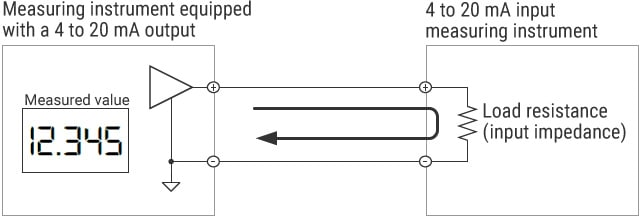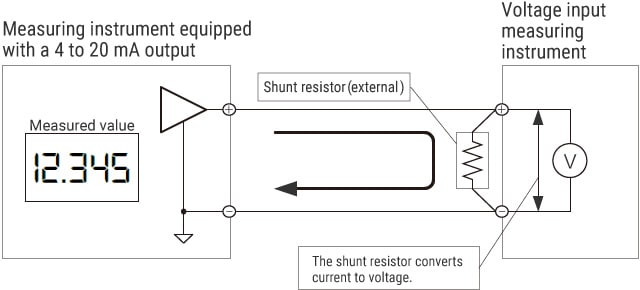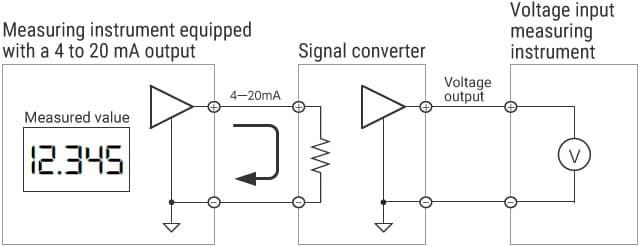# Measurement Methods for Single Connection

Methods for current measurement (4 to 20 mA) vary depending on how the 4 to 20 mA target output device is connected to one or more 4 to 20 mA input devices.
This section explains measurement methods when each input device and output device is connected in a one-to-one manner (single connection). There is a method using a 4 to 20 mA input measuring instrument and another method using a shunt resistor. This section also introduces measurement principles and key points in selection for each of these methods.

## Measurement method using an instrument equipped with a 4 to 20 mA input

When using an instrument that has an input range of 4 to 20 mA, measurement can be performed through direct connection.

WiringKey point in selection

• Satisfy the relationship of “maximum load resistance of the 4 to 20 mA output > load resistance of the 4 to 20 mA input.” If this relationship is not satisfied, the 4 to 20 mA output circuit cannot operate properly. This issue will lead to measurement errors.

## Measurement method using a shunt resistor to convert current to voltage

When using an instrument that has a voltage input range, the user can perform measurement by externally connecting a shunt resistor to convert current to voltage. The current to voltage conversion is carried out as follows: the current that flows through a shunt resistor is converted to voltage through Ohm’s Law (E = I × R).

WiringKey points in selection

• Satisfy the relationship of “maximum load resistance of the 4 to 20 mA output > resistance value of the shunt resistor.” If this relationship is not satisfied, the 4 to 20 mA output circuit cannot operate properly. This issue will lead to measurement errors.
• For details on how to select a shunt resistor, refer to “How to Select a Shunt Resistor.”

## Measurement method using a signal converter

By using a signal converter, you can measure a 4 to 20 mA output with an instrument that has a voltage input range. You can select your ideal voltage output range from our lineup of signal converters.
It is also possible to insulate an instrument by using an insulation-type signal converter.

WiringKey points in selection

• Satisfy the relationship of “maximum load resistance of the 4 to 20 mA output > load resistance of the signal converter.” If this relationship is not satisfied, the 4 to 20 mA output circuit cannot operate properly. This issue will lead to measurement errors.
• If a potential difference occurs between the GNDs of each channel when measuring multiple measurement targets, you cannot use an instrument that shares internal GNDs. In this case, use an insulation-type signal converter.

INDEX